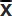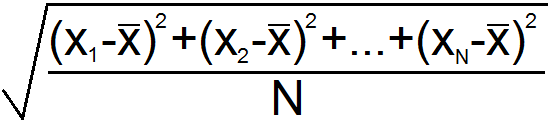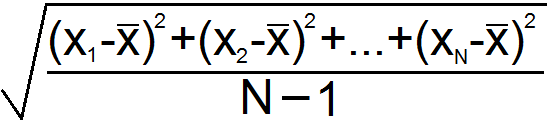# How to Compute Mean, Median, Mode, Range, and Standard Deviation

In statistics and data analysis, the mean, median, mode, range, and standard deviation tell researchers how the data is distributed. Each of the five measures can be calculated with simple arithmetic. The mean and median indicate the "center" of the data points. The mode is the value or values that occur most frequently. Range is the span between the smallest value and largest value. Standard deviation measures how far the data "deviates" from the center, on average. Knowing how to calculate these statistical measures will help you analyze data from surveys and experiments.

## Mean

The arithmetic mean or average of a set of numbers is the expected value. The mean is calculated by adding up all the values, and then dividing that sum by the number of values.

For example, suppose a teacher has seven students and records the following seven test scores for her class: 98, 96, 96, 84, 80, 80, and 72. The average test score is

(98+96+96+84+81+81+73)/7 = 609/7 = 87.

If one more student entered her class and took the test, the expected score would be an 87.

## Median

The median is the middle value in a set of values. To find the median, order the numbers from largest to smallest, and then choose the value in the middle. For example, consider the following set of nine numbers:

10, 13, 4, 25, 8, 12, 9, 19, 18

If we arrange them in descending order, we get

25, 19, 18, 13, 12, 10, 9, 8, 4

The middle value is 12, so the median = 12. What if we have a set with an even number of values? For example, consider the set

1, 2, 3, 4, 5, 6.

Both 3 and 4 are in the middle. In this case, we must take the average of the two middle numbers. Since (3+4)/2 = 3.5, the median = 3.5.

## Mode

The mode of a set is the value or values that occur most frequently. There can be more than one mode in a set. If there is more than one mode, you simply list all of the modes; you do not have to average them. For example, consider the set

10, 10, 4, 8, 10, 8, 3, 9, 14

The number 10 occurs three times, and no other numbers occur as frequently. Therefore, the mode = 10

Now consider this set

10, 10, 4, 8, 10, 8, 3, 8, 14

Both 10 and 8 occur three times each, and no other numbers occur as often. Therefore, the modes are 8 and 10.

## Range

The range of a set of numbers is the maximum distance between any two values. In other words, it's the difference between the largest and smallest values. Knowing the range gives you an idea of how close together the data points are. For example, consider the set of test scores

78, 88, 67, 90, 92, 83, 97

The highest test score is 97 and the lowest is 67, therefore the range is 97-67 = 30.

## Standard Deviation

The standard deviation is another way to measure how close together the elements are in a set of data. The s.d. is the average distance between each data point and the mean. Knowing the standard deviation gives a more complete picture of the distribution of elements in a data set. Suppose you have N data points and you label them X1, X2, X3,... XN, and you call the mean. There are two formulas for standard deviation depending on whether your data is a complete set, or a sample take from a larger set.

For example, suppose your data is all of the ACT scores of the students in a small class. Then the standard deviation formula isSuppose the scores are 15, 21, 21, 21, 25, 30, and 35. The mean of this set is 24. The s.d. is

sqrt[((15-24)2+(21-24)2+(21-24)2+(21-24)2+(25-24)2+(30-24)2+(35-24)2)/7]
= sqrt[266/7]
= sqrt
= 6.16

If you take a random sample of ACT scores from a large school, the standard deviation formula isFor example, suppose you select ten students at random from a high school, and their ACT scores are 17, 20, 24, 25, 26, 26, 29, 29, 30 and 32. The average of this set is 25.8. The standard deviation is

sqrt[((17-25.8)2+(20-25.8)2+(24-25.8)2+(25-25.82+(26-25.8)2
+(26-25.8)2+(29-25.9)2+(29-25.8)2+(30-25.8)2+(32-25.8)2)/(10-1)]
= sqrt[(191.6 )/(10-1)]
= sqrt[191.6/9]
= sqrt[21.2889]
= 4.61